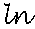Search IntMath
Close

# 5. Natural Logarithms (to the base e)

by M. Bourne

The number e frequently occurs in mathematics (especially calculus) and is an irrational constant (like π). Its value is e = 2.718 281 828 ...

Apart from logarithms to base 10 which we saw in the last section, we can also have logarithms to base e. These are called natural logarithms.

We usually write natural logarithms using ln, as follows:

ln x to mean log_e x (that is, "log x to the base e")

Natural logarithms are commonly used throughout science and engineering. (For example, see Applications of Derivatives of Logarithms.)

Where does this value "e" come from? Go to Calculating the Value of e to find out.

## NotationNOTE: Please don't write natural log as

"In" (as in "She lives IN Singapore.")

Make sure it is

"" (as in L for logarithm and N for natural).

I know it looks like "In" on your calculator because of the font they use, but you only confuse yourself if you don't write it properly.

Actually, the ln notation confuses a lot of students and it would be better if we (and calculators) wrote it our in full. That is log_e.

### Example 1

Find the natural logarithm of 9.178.

This means "Find log_e 9.178", which we can also write as "Find ln 9.178".

Using our calculator, we get

ln 9.178 = 2.2168

Check: Using the definition of a logarithm, we check as follows: 2.718\ 281\ 828 ^2.2168 = 9.1781.

It checks OK.

## Change of Base

At times we need to change from one base to another. The change of base formula (to change from base a to base b) is as follows:

log_b x=(log_a x)/(log_a b)

### Example 2

Find the value of log_3 8.7.

[This problem is the same as answering: 3^?= 8.7].

We cannot find log_3 8.7 on a calculator. We need to use the change of base formula.

In this case, b = 3 and x = 8.7.

So

log_3 8.7=(log_10 8.7)/(log_10 3) =0.9395192/0.4771212 =1.9691414

CHECK: By calculator, 3^1.9691414 = 8.6999989.

[We could have used natural logs as well, log_3 8.7=(ln 8.7)/(ln 3) which will give us the same answer.]

### Exercises

1. Use logarithms to base 10 to find log_2 86.

We estimate an answer in the range: 6 to 7, because 2^6=64 and 2^7=128, and 86 is between these 2 values.

log_2 86 = (log 86)/(log 2) =1.934498451/0.301029995 =6.426264755

Our answer is between 6 and 7, as expected.

2. Find the natural logarithm of 1.394.

ln 1.394 = 0.332177312

Check: This means e^0.332177312=1.394

See also the Interactive Log Table where you can easily find log values to different bases.

## Application of Exponential FunctionsHere is an exponential graph which made lots of people very RICH (as long as they sold out at the peak).

Check out the Dow Jones Industrial Average graph.

## Problem SolverThis tool combines the power of mathematical computation engine that excels at solving mathematical formulas with the power of GPT large language models to parse and generate natural language. This creates math problem solver thats more accurate than ChatGPT, more flexible than a calculator, and faster answers than a human tutor. Learn More.